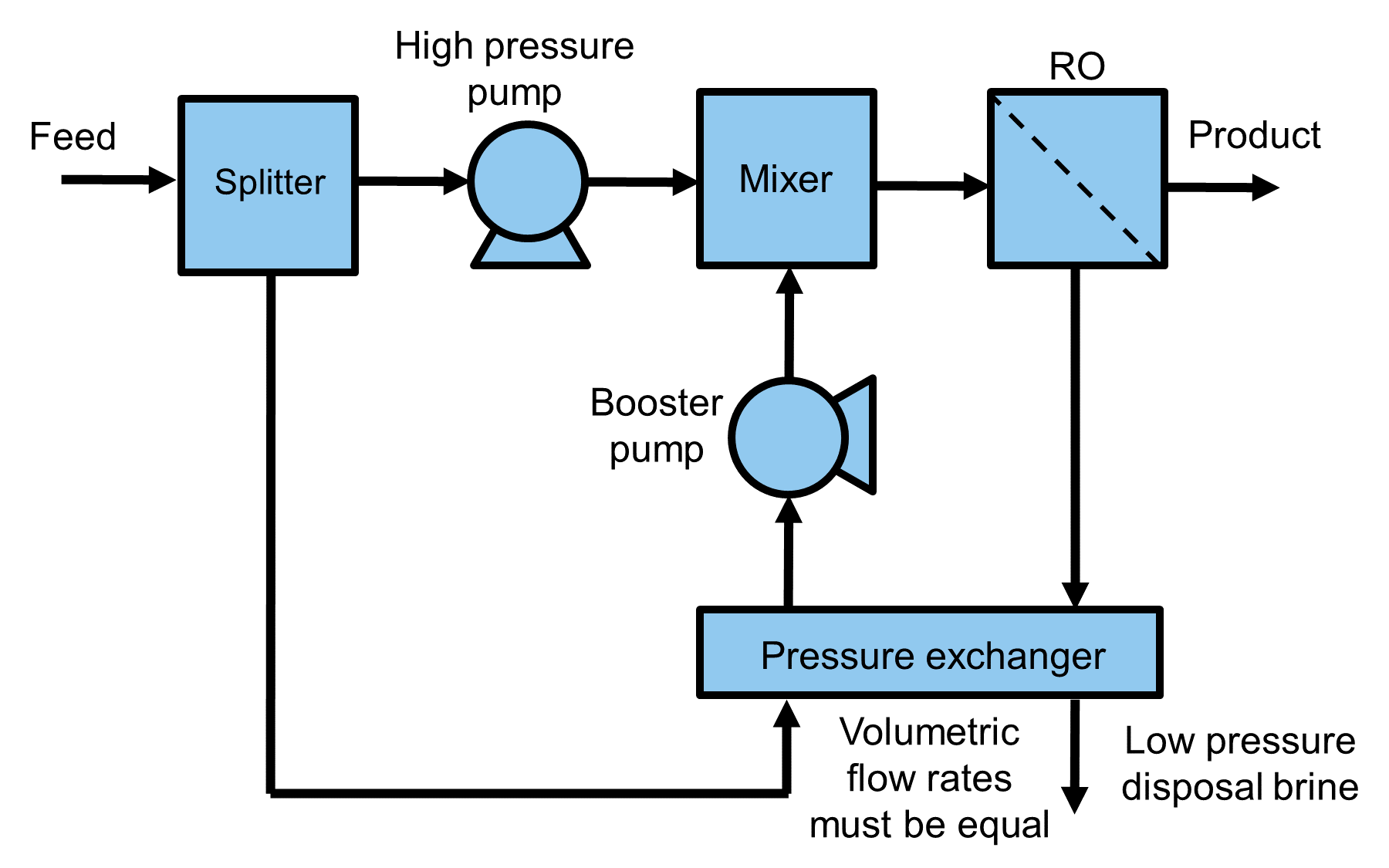# Pressure Exchanger

This pressure exchanger unit model:
• is 0-dimensional

• is isothermal

• supports a single liquid phase only

• assumes no mixing or leakage between the low and high pressure side

• assumes equal flowrates on both sides

## Degrees of Freedom

Generally, pressure exchangers are not used in isolation and are part of an energy recovery system that includes a mixer, splitter, and a booster pump, as shown in Figure 1. This energy recovery system only adds one degree of freedom to the overall model.

Typically, the following variables are fixed to fully specify the energy recovery system:
• the pressure exchanger efficiency (efficiency_pressure_exchanger located on the pressure exchanger unit model)

• the booster pump efficiency (efficiency_pump located on the pump unit model)

Where the system is also subject to following constraints:
• volumetric flowrate is equal on both sides of the pressure exchanger (constraint is included in pressure exchanger unit model)

• booster pump matches the high pressure pump outlet pressure (constraint must be added by the user or at the mixer with the equality momentum mixing type option)Figure 1. Schematic representation of an energy recovery system using a pressure exchanger.

## Model Structure

The pressure exchanger model consists of 2 ControlVolume0DBlocks: one for the low-pressure side and high-pressure side.

## Sets

Description

Symbol

Indices

Time

$$t$$



Phases

$$p$$

[‘Liq’]

Components

$$j$$

[‘H2O’, ‘NaCl’]*

*Solute depends on the imported property package; example shown here is for the NaCl property model.

## Variables

The pressure exchanger unit model includes the following variables:

Description

Symbol

Variable Name

Index

Units

Pyomo Type

Efficiency

$$\eta$$

efficiency_pressure_exchanger

[t]

$$\text{dimensionless}$$

Var

Each control volume (i.e. low_presssure_side, and high_pressure_side) has the following variables of interest:

Description

Symbol

Variable Name

Index

Units

Pyomo Type

Pressure change

$$ΔP$$

deltaP

[t]

*

Var

Work

$$W$$

work

[t]

*

Expression

*Units depends on the imported property package

Each property block on both control volumes (i.e. properties_in and properties_out) has the following variables of interest:

Description

Symbol

Variable Name

Index

Units

Pyomo Type

Volumetric flowrate

$$Q$$

flow_vol

None

*

Var

Temperature

$$T$$

temperature

[t]

*

Var

Pressure

$$P$$

pressure

[t]

*

Var

*Units depends on the imported property package

## Equations

Description

Equation

Mass balance for each side

$$M_{out, j} = M_{in, j}$$

Momentum balance for each side

$$P_{out} = P_{in} + ΔP$$

Isothermal assumption for each side

$$T_{out} = T_{in}$$

Equal volumetric flowrate*

$$Q_{LPS} = Q_{HPS}$$

Pressure transfer*

$$ΔP_{LPS} = - \eta ΔP_{HPS}$$

* LPS stands for low pressure side, HPS stands for high pressure side